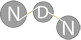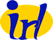NS-3 based Named Data Networking (NDN) simulator ndnSIM 2.0: NDN, CCN, CCNx, content centric networks API Documentation
ndn::random Namespace Reference

## Functions

static CryptoPP::AutoSeededRandomPool & getSecureRandomGenerator ()

uint32_t generateSecureWord32 ()
Generate a cryptographically secure random integer from the range [0, 2^32) More...

uint64_t generateSecureWord64 ()
Generate a cryptographically secure random integer from the range [0, 2^64) More...

static boost::random::mt19937 & getRandomGenerator ()

uint32_t generateWord32 ()
Generate a cryptographically non-secure random integer from the range [0, 2^32) More...

uint64_t generateWord64 ()
Generate a cryptographically non-secure random integer from range [0, 2^64) More...

## Function Documentation

 static CryptoPP::AutoSeededRandomPool& ndn::random::getSecureRandomGenerator ( )
static

Definition at line 38 of file random.cpp.

Referenced by generateSecureWord32(), and generateSecureWord64().

 uint32_t ndn::random::generateSecureWord32 ( )

Generate a cryptographically secure random integer from the range [0, 2^32)

This method uses CryptoPP routines

Definition at line 46 of file random.cpp.

References getSecureRandomGenerator().

 uint64_t ndn::random::generateSecureWord64 ( )

Generate a cryptographically secure random integer from the range [0, 2^64)

This method uses CryptoPP routines

Definition at line 52 of file random.cpp.

References getSecureRandomGenerator().

 static boost::random::mt19937& ndn::random::getRandomGenerator ( )
static

Definition at line 64 of file random.cpp.

Referenced by generateWord32(), and generateWord64().

 uint32_t ndn::random::generateWord32 ( )

Generate a cryptographically non-secure random integer from the range [0, 2^32)

This method uses Boost.Random routines

This version is faster than generateSecureWord32, but it should not be used when cryptographically secure random integers are needed (e.g., when creating signing or encryption keys)

Definition at line 73 of file random.cpp.

References getRandomGenerator().

 uint64_t ndn::random::generateWord64 ( )

Generate a cryptographically non-secure random integer from range [0, 2^64)

This method uses Boost.Random routines

This version is faster than generateSecureWord64, but it should not be used when cryptographically secure random integers are needed (e.g., when creating signing or encryption keys)

Definition at line 80 of file random.cpp.

References getRandomGenerator().